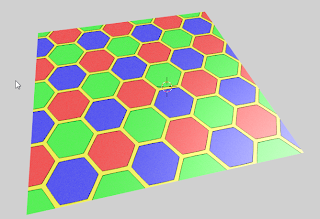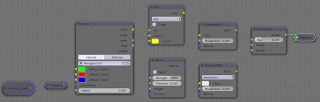### A hexagon shader in OSL, second edition

A while ago I made a simple OSL shader for hexagonal tilings. Prompted by some questions on BlenderArtists I decided to create a more versatile version that retained the color options but added the distance to the closest edge. That feature may help in creating crips edge patterns because the previously availble distance to the closest center creates rounded shapes that might be suitable for bee hives but not for hard edged stuff like floor tiles etc.The noodle used to create the image is shown below (click to enlarge):The color code has stayed the same except for the calculation of the distance to the edge. This might be a bit inefficient, but at least it's easy to read.
The additions are shown below, the full code is available on GitHub.
```    // distance to nearest edge

float x = mod(Coordinates/3,1.0);
float y = mod(Coordinates/3,A2);

#define N 18
vector hc[N] = {
vector(  0, -A2/3     ,0),
vector(  0,     0     ,0),
vector(  0,  A2/3     ,0),
vector(  0,2*A2/3     ,0),
vector(  0,  A2       ,0),
vector(  0,4*A2/3     ,0),

vector(0.5, -A2/3+A2/6,0),
vector(0.5,     0+A2/6,0),
vector(0.5,  A2/3+A2/6,0),
vector(0.5,2*A2/3+A2/6,0),
vector(0.5,  A2  +A2/6,0),
vector(0.5,4*A2/3+A2/6,0),

vector(1.0, -A2/3     ,0),
vector(1.0,     0     ,0),
vector(1.0,  A2/3     ,0),
vector(1.0,2*A2/3     ,0),
vector(1.0,  A2       ,0),
vector(1.0,4*A2/3     ,0)
};

float d[N], t;

for(int i=0; i < N; i++){
float dx = x - hc[i];
float dy = y - hc[i];
d[i] = hypot(dx, dy);
}

for(int j= N-1; j >= 0; j--){
for(int i= 0; i < j; i++){
if(d[i] > d[i+1]){
SWAP(t, d[i], d[i+1]);
}
}
}

Center  = d;
Edge    = d - d;
InEdge  = Edge < Width;```
The approach we have taken is very simple: the `hc` enumerates all nearby hexagon centers. We then calculate all the distances to these points and sort them shortest to longest (yes with a bubble sort: with 18 elements it might just be faster to do it with a more efficient sorting algorithm at the cost of much more complex code so I don't bother).
The `Edge` is not realy the distance to the closest edge but the difference between the closest center and the next closest. Near the edge these values are more and more the same so `Edge` will approach zero. For convience we provide a comparison with some threshold also.
If you would like to know more about programming OSL you might be interested in my book "Open Shading Language for Blender". More on the availability of this book and a sample can be found on this page.